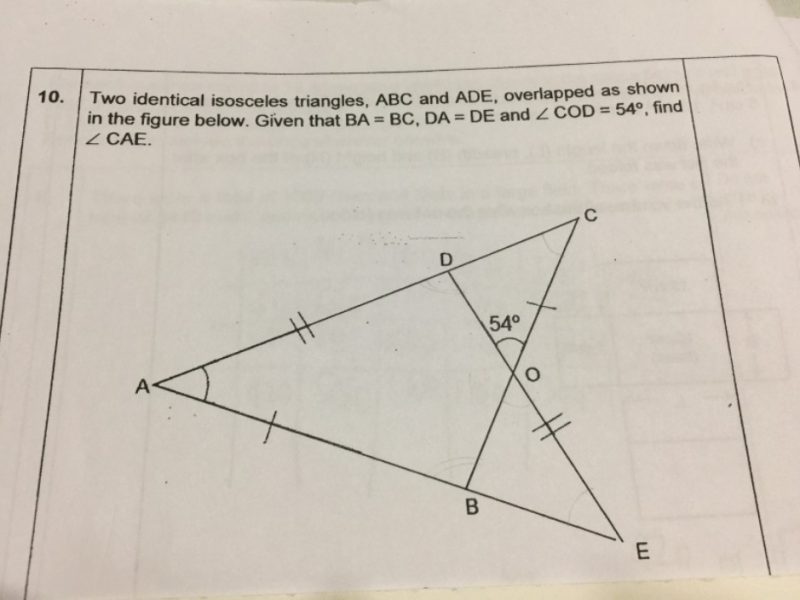# QuestionCan’t get 42deg

Let Angle CAE = x.

Then angle ADE = 180 – 2x.

Consider triangle ODC.

180 – 2x = 54 + x (exterior angle is the sum of the opposite interior angles)

x = 42 degrees

0 Replies 0 Likes ✔Accepted Answer